# GED Math : Volume of a Rectangular Solid

## Example Questions

← Previous 1

### Example Question #1 : Volume Of A Rectangular Solid

An aquarium takes the shape of a rectangular prism 60 centimeters high, 60 centimeters wide,  and 120 centimeters long. One-fourth of a cubic meter of water is poured into the aquarium after it has been emptied. How much more water can it hold?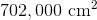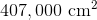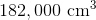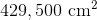Explanation:

One cubic meter of water is equal to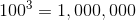cubic centimeters; one-fourth of a cubic meter is 250,000 centimeters. The volume of the aquarium is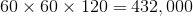cubic centimeters.

Therefore, after having one-fourth of a cubic meter of water poured in, there is room left for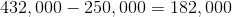cubic centimeters of water.

### Example Question #2 : Volume Of A Rectangular Solid

A large aquarium has a rectangular base nine meters square and is ten and one-half meters high. The eight inlet pipes used to fill the aquarium does so at a rate of 200 liters per minute each. To the nearest hour, how long does it take for all eight pipes working together to fill the aquarium to 80% capacity?

You will need the conversion factor 1 liter = 1,000 cubic centimeters.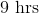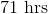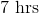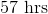Explanation:

The aquarium is a rectangular prism with dimensions 9 meters by 9 meters by 10.5 meters, each of which can be converted to centimeters by multiplying by 100. So the dimensions are 900 cm x 900 cm x 1,050 cm, and the volume is the product of the three, or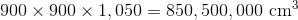or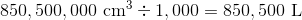80% of this is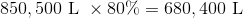Each pipe fills the aquarium at 200 L per minute, so the eight pipes working together fill it at a rate of 1,600 L per minute. Divide, and the aquarium is filled at 80% capacity in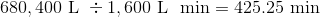In hours, this is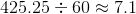so 7 hours is the correct response.

### Example Question #3 : Volume Of A Rectangular Solid

If a swimming pool is rectangular, and its base area is 20 feet squared, what is the volume if the height of the pool is 8 feet?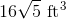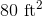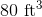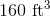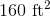Explanation:

The volume of the rectangular solid can be written as: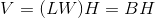The length times width constitutes the base of the rectangular solid, and is given in the question.

Substitute the known dimensions into the formula.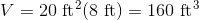The answer is:### Example Question #4 : Volume Of A Rectangular Solid

A rectangular wood block has the dimensions of 6 inches, 1 foot, and 8 inches.  What is the volume in feet?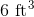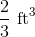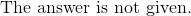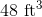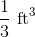Explanation:

Convert all the dimensions into feet.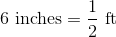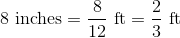Write the formula for the volume of the block.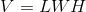Substitute the dimensions and solve for volume.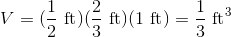The answer is:### Example Question #5 : Volume Of A Rectangular Solid

Find the volume of a rectangular wood block with a length of, width of, and a height of.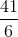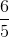Explanation:

Write the formula for the volume of a rectangular prism.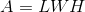Substitute the dimensions into the formula.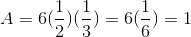The answer is:### Example Question #1 : Volume Of A Rectangular Solid

Determine the volume of a brick in inches if its dimensions are 3 inches by 6 inches by 2 inches.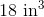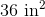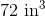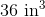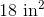Explanation:

The volume of a rectangular prism can be represented as:Substitute the dimensions into the formula.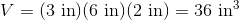Be sure that inches is cubed for volume.

The answer is:### Example Question #7 : Volume Of A Rectangular Solid

What is the volume of a brick with a length of 6 inches, width of 4 inches, and a height of 3 inches?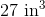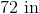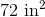Explanation:

The volume of a brick is similar to finding the volume of a rectangular solid.

The volume for a rectangular solid is:Substitute the dimensions.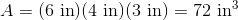The answer is:### Example Question #1 : Volume Of A Rectangular Solid

Find the volume of the rectangular solid with a length, width, and height of 4,5,and 6, respectively.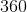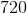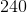Explanation:

Write the volume for the rectangular solid.Substitute the dimensions into the formula.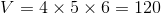The answer is:### Example Question #2 : Volume Of A Rectangular Solid

Find the volume of a rectangular solid if the length, width, and height are 6, 12, and 20, respectively.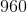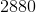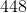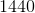Explanation:

Write the volume for a rectangular solid.Substitute the dimensions into the formula.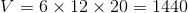The answer is:### Example Question #10 : Volume Of A Rectangular Solid

What is the volume of a book with a length of 7 inches, width of 4 inches, and a height of 1 inches?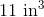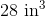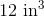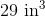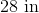Explanation:

The book resembles a rectangular solid.  Write the formula for the volume of a rectangular solid.Substitute the dimensions into the equation.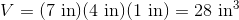The answer is:← Previous 1